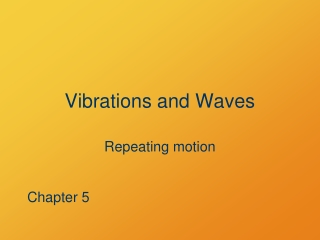DownloadDownload PresentationVibrations and Waves

# Vibrations and Waves

Télécharger la présentation## Vibrations and Waves

- - - - - - - - - - - - - - - - - - - - - - - - - - - E N D - - - - - - - - - - - - - - - - - - - - - - - - - - -
##### Presentation Transcript

1. Vibrations and Waves Repeating motion Chapter 5

2. Vibrations Just back and forth pp. 104–106

3. compression tension Structure of Solids • Atoms and molecules connected by chemical bonds • Considerable force needed to deform

4. small stretch larger stretch Elasticity of Solids Small deformations are proportional to force Hooke’s Law: uttensio, sic vis(as the pull, so the stretch) Robert Hooke, 1635–1703

5. Restoring Force • A Hooke’s law force always draws the system back to equilibrium • If you push or pull a mass on a spring away from equilibrium, how will it move after release?

6. Oscillation • Spring oscillations are repeating motion • So is uniform circular motion • Circular motion concepts are useful in describing any repeating motion • Cycle • Period and frequency • Phase angle • Trigonometric functions

7. Oscillation amplitude y time period

8. Oscillation Terms • Period = duration of one repeat • Frequency = repeats per unit time • Unit hertz = Hz = cycle/s • Amplitude = maximum excursion from equilibrium

9. Period • Stiffness of spring • Mass of load • Not amplitude Determining factors

10. L q m Simple Pendulum • Restoring force • Approximately Hooke’s law • Determining factors • length L • Gravitational field g • Not mass m!

11. Waves Travel in space pp. 106–121

12. Waves and Vibrations • Vibrations: Repeat in time • no net displacement of disturbance • no net displacement of medium • Waves: Repeat in time and space • wave travels • no net displacement of medium

13. Wave Pulse in a Rope • Why does the pulse move? • What determines its speed? • What is happening inside the rope?

14. a c b d What are the velocity and acceleration of the string particles at the following positions? Why? • middle (leading edge) • crest • middle (trailing edge) • trough Points to Ponder The particles of the string change their motion as the wave travels. What force accelerates them?

15. crest l l trough Features of a Wave • Crest: high point • Trough: low point • Wavelength: crest-crest distance (m) • Period: crest-crest-timing (s)

16. v A Features of a Wave • AmplitudeA: (crest height – trough height) / 2 • Frequencyf: repeats in a given time (cyc/s = Hz) • Velocityv: speed of crest motion (m/s)

17. Relations between Features • Period T = 1 / f; Frequency f = 1 / T • Velocity v = l / T = lf • Wavelengthl=vT=v / f • Frequencyf = v / l; Period T = l / v

18. Wave Pulse in a Slinky • Why does the pulse move? • What determines its speed? • What is happening inside the spring?

19. Types of Waves • Motion of the medium is perpendicular to the direction the wave travels: transverse wave (example: string wave) • Motion of the medium is parallel to the direction the wave travels: longitudinal wave (examples: sound wave, slinky wave) • Animation

20. Wave Refraction Direction changes to keep wave front continuous Source: University of Southampton, Institute for Sound and Vibration Research

21. Example u = 1/2 u = 3/4 u = 1

22. Ocean Wave Refraction

23. Combining Waves

24. result Adding Waves Together 3 0 –3

25. Wave Interference • Constructive: Sum of waves has increased amplitude • Destructive: Sum of waves has decreased amplitude • Two-wave simulation

26. Wave Refraction Direction changes to keep wave front continuous Source: University of Southampton, Institute for Sound and Vibration Research

27. Beats • Waves of similar frequency combine to give alternating times of constructive and destructive interference

28. 2-D Wave Interference • interference patterns • http://www.falstad.com/ripple/

29. Standing Waves • Add a wave to an identical wave traveling in the opposite direction • (Its reflection) • Medium vibrates, but waves don’t travel • Nodes: positions of zero variation • Antinodes: positions of maximum variation

30. Sound Longitudinal wave in 3 dimensions pp. 111–121

31. Pipes: “Closed” and “Open” Tube Modes Source: Halliday, Resnick, and Walker, Fundamentals of Physics, 2003, p 419.

32. Harmonic Sequence • Western musical scale and harmonies are based on overtone series

33. Doppler Effect Distance and time § 16.9

34. Moving Source or Detector • Source: successive wave fronts do not emanate from the same place • Detector: successive wave fronts are not detected at the same place • Simulation: http://physics.bu.edu/~duffy/HTML5/doppler.html

35. vD vS v 3 2 1 0 D Moving Source or Detector

36. Two-Dimensional Waves • Ocean waves, earthquake surface waves • Wave types animations • Membrane standing waves animations

37. Water Waves • Restoring force is gravity • Fastest in deep water, slow in shallow water • Breaking and refractive behavior in shallow water • Speed depends on wavelength • Very complicated behavior!

38. Shoaling and Breaking • Waves slow in shallows by shore • Wavelength shortens and amplitude rises • Troughs move slower than crests • Crests fall forward

39. Example u = 1/2 u = 3/4 u = 1

40. Ocean Wave Refraction